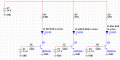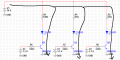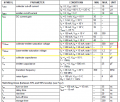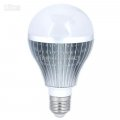# Controlling RGB Led using PWM of Microcontroller

#### BramLabs

Joined Nov 21, 2013
98
Sir, i want to ask. I have a project to control 11 LED RGB using microcontroller.
The circuit is as you can see below :The RGB Led i'm using with is SMD 5050 RGB. Or you can see the datasheet in the attachment file.

LED 1 = Red LED
LED 2 = Blue LED
LED 3 = Green LED

So basically, the total of the RGB LED SMD 5050 is 11 LED i'm using with because in 1 package of IC, contains red, green, and blue.

3 of them are in 1 IC led package.

And the specification is :
Red LED needs Vf = 2,1V, If = 20mA ;
Green LED needs Vf = 3,2V, If = 20mA ;
Blue LED needs Vf = 3,2V, If = 20mA ;

So at the green LED and blue LED, i use 25 ohm resistor.
This is because :
36 Volt - 11*(3,2V) - 0.3V (assume when the 2N222A transistor or in reality, i'm using MMBT2222A, is in saturation region ) / 20 mA = 25 ohm

And at RED LED, i use 630 ohm resistor, because :
36 Volt - 11*(2,1V) - 0.3V (assume when the 2N222A transistor or in reality, i'm using MMBT2222A, is in saturation region) / 20 mA = 630 ohm.

What i want to ask is that, AT THE RED LED circuit, when i applied KVL equation, 36 Volt - 11*(2,1V) - 0,3V = 12.6V ( the transistor is in the saturation region ). Where the 12.6 V will be dissipated ? Will it be dissipated at the resistor of 630 ohm ? Or will it be dissipated at the transistor MMBT2222A ? My guess is the rest of the voltage ( 12.6 V ) will be dissipated on the resistor. So, i need at least P = 12.6V * 20mA = 0.252 Watt resistor ( i think i'm going to use 1/2 Watt resistor )
Am i right sir ?

Thanks before.

#### Attachments

• 276.8 KB Views: 8
• 127.3 KB Views: 1
Last edited:

#### Picbuster

Joined Dec 2, 2013
1,040
Hi Bramlabs,
This schematic is, as far as I can see, ceiling destroying.
The current that will run = 36-(vf+Vbc in saturation) in your case (36- 4 approx.)/25 = 1,28 Amp.
The resistor will carry 1.28^2 *25 =41 Watt approx.

Please remember that a led is not limiting its current but will work within the spec's at that current.

please recalculate with all the above in mind.

•BramLabs

#### BramLabs

Joined Nov 21, 2013
98
Hi Bramlabs,
This schematic is, as far as I can see, ceiling destroying.
The current that will run = 36-(vf+Vbc in saturation) in your case (36- 4 approx.)/25 = 1,28 Amp.
The resistor will carry 1.28^2 *25 =41 Watt approx.

Please remember that a led is not limiting its current but will work within the spec's at that current.

please recalculate with all the above in mind.
Sir, i think when in saturation region, the transistor will work like an on switch. And when this happened we should calculate the VCE not VCB or VBC. And as i can see from the datasheet, i found that VCE in saturation costs me 0.3 V.
So basically, when the switch ( transistor ) is on, the current will go through like this :So, if we calculate, ( 36V - 11 Red LED * Vf which in this case the Vf is 2,1V - 0.3V as the result VCE when it's in saturation region ) / 20mA current needed ( because in series circuit, the current goes through it, will all be the same, so because i need 20 mA to make the LED work then, i need 20 mA that flow through that circuit )

And also, if you check in the datasheet, you'll see also, the VCB when the emitter is in open condition, it can holds up to 75 V maximum. The current won't go through collector to base, because the current only can flow to collector to emitter ( Ie = Ic + Ib ), not collector to base. The current through the collector is maintained by the current through the base.

Last edited:

#### ebeowulf17

Joined Aug 12, 2014
3,307
Your original schematic doesn't indicate 11 RGB LEDs, but instead only one. I think this is the source of confusion. I initially assumed you were going to repeat the circuit above (in parallel) 10 more times.

If, on the other hand, all 11 LEDs are in series, right at the point in your schematic where the single LED is shown, then I think your approach is correct.

And yes, you'll need a high power resistor on the red leg! I'm no expert, but your calculations look right to me.

•BramLabs

#### BramLabs

Joined Nov 21, 2013
98
Your original schematic doesn't indicate 11 RGB LEDs, but instead only one. I think this is the source of confusion. I initially assumed you were going to repeat the circuit above (in parallel) 10 more times.

If, on the other hand, all 11 LEDs are in series, right at the point in your schematic where the single LED is shown, then I think your approach is correct.

And yes, you'll need a high power resistor on the red leg! I'm no expert, but your calculations look right to me.
Woah, really sir, i'm really-really sorry if i confused all of you. Yeah, i connect all the 11 LED in series. Really big thanks for your correction sir ^^. So, in the RED RGB resistor, i should at least use 1/2 Watt of resistor based on my calculation, am i right sir ? Thank you before ^^

#### ebeowulf17

Joined Aug 12, 2014
3,307
That sounds right to me (although, like I said, I'm new at all of this, so I could be wrong!)

•BramLabs

#### BramLabs

Joined Nov 21, 2013
98
That sounds right to me (although, like I said, I'm new at all of this, so I could be wrong!)
Okay sir, i really appricate your answerBig thanks to you ! At least my design not really bad at all. ahahaha... Because you think that it's right >_<v

Thank you sir !

#### ebeowulf17

Joined Aug 12, 2014
3,307
Oops! Reading more closely, I see now that there were notes on the LEDs in the schematic indicating that they represented 11 in series. I overlooked that earlier. Sorry.

•BramLabs

#### BramLabs

Joined Nov 21, 2013
98
Oops! Reading more closely, I see now that there were notes on the LEDs in the schematic indicating that they represented 11 in series. I overlooked that earlier. Sorry.
Oh no sir, you were right ! I just edited my thread earlier about 1 hour ago. ahahaahaha... So i added the note ( 11 red rgb in series, 11 green rgb in series and 11 blue rgb in series ).
Thank you before for your concern !

#### ScottWang

Joined Aug 23, 2012
7,306
Hi Bramlabs,
This schematic is, as far as I can see, ceiling destroying.
The current that will run = 36-(vf+Vbc in saturation) in your case (36- 4 approx.)/25 = 1,28 Amp.
The resistor will carry 1.28^2 *25 =41 Watt approx.

Please remember that a led is not limiting its current but will work within the spec's at that current.

please recalculate with all the above in mind.
Will you calculate from V1, current limiting resistor, Led (11 pcs) and Vce to see how the three answers are?

#### ScottWang

Joined Aug 23, 2012
7,306
Your three Rb are too small.
How is the maximum duty cycle of the pwm? (this will be related with R1, R2, R4)

•BramLabs

#### BramLabs

Joined Nov 21, 2013
98
Your three Rb are too small.
How is the maximum duty cycle of the pwm? (this will be related with R1, R2, R4)
Hi sir,
Hmm.... you mean the 330 ohm sir?
If i calculate the voltage from microcontroller digital pin ( 3,3 volt ). The calculation will be like this :

Vmcu - Vbe / 330 ohm = Ib
3.3V - 0.7V ( i assume the Vbe is 0.7v ) / 330 ohm = 7.8 mA

And if i looked at the datasheet ( i'm using the SMD version of 2N222A, that's MMBT2222A ) :The red rectangular above, indicates that if i have Ib around 15 mA, with Vce = 0.3 V, i will get Ic up to 150 mA.
And from my calculation before, i got Ib = 7.8 mA.
And because i just need 20 mA of Ic to turned all of the 11 LED in series ON, i think 7.8 mA which is because i use 330 ohm resistor on the base of transistor, is not enough for me to get 150 mA, but enough to get 20 mA ( the current that flow through the collector, depends on the Load also. In my case, my loads are the resistor 630 ohm at the red LED RGB or 25 ohm at the green / blue LED RGB AND ALSO the 11 red LED RGB or 11 green / blue LED RGB ).
If i'm using more than 330 ohm, just say 1k ohm, i'll get :

Ib = 3.3v - 0.7v ( i assume the Vbe is 0.7v ) / 1k ohm = 2.6 mA
Then, it means i get less current of Ib and i'll also get less current of Ic.
Why should i increase the resistor on Rb like you said ?

And for your question how maximum duty cycle of PWM i'm using with, i think i'll use 100% PWM for making a white shining light. Because 100% pwm for RED, GREEN, and BLUE, with the CORRECT RESISTOR, will give us white light. And if the red, green, and blue LED RGB didn't have a correct resistor, the light maybe white but not really white, i think it's blueish white.

Thank you before sir for help !
I really appreciate it.

#### Attachments

• 127.3 KB Views: 0
• 92.6 KB Views: 0
Last edited:

#### ScottWang

Joined Aug 23, 2012
7,306
In the table you showed as Ic = 150 mA, Ib = 15 mA, hFe = 150 mA/15 mA = 10.
The hFe should be counting as 10 when the bjt in the saturation region, so you have to calculate from Ic = 20mA, and then how is Ib?

The duty cycle will be affecting the values of Ic, Ib, normally when the led just lighting up then it can be calculated as 20 mA, if you using the max cycle of pwm is 80% or 90% then the Ic can be exceed 20 mA, you also need to recalculate the R1 and R2 for Ic, it is different with my calculation.

You recalculate for Ic from V1, current limiting resistor, Led (11 pcs) and Vce

•BramLabs

#### BramLabs

Joined Nov 21, 2013
98
In the table you showed as Ic = 150 mA, Ib = 15 mA, hFe = 150 mA/15 mA = 10.
The hFe should be counting as 10 when the bjt in the saturation region, so you have to calculate from Ic = 20mA, and then how is Ib?

The duty cycle will be affecting the values of Ic, Ib, normally when the led just lighting up then it can be calculated as 20 mA, if you using the max cycle of pwm is 80% or 90% then the Ic can be exceed 20 mA, you also need to recalculate the R1 and R2 for Ic, it is different with my calculation.

You recalculate for Ic from V1, current limiting resistor, Led (11 pcs) and Vce
Hmm....
So should i think from the table that i use hfe = 10 ?
So if i apply hfe = 10, then :
Ic i want to use = 20 mA
Ib = Ic / hfe = 20 mA / 10 = 2 mA
Then Rb = 3.3v - 0.7v / 2 mA = 1300 ohm

Am i right sir ?

Oh yeah sir, about the If of the RGB LED. If i looked on the datasheet ( i already attached it on my first post this thread ), it says that If = 20 mA. I don't know whether it's maximum or the current that will flow if Vf is required or it's just the normal current if Vf is required.

And you asked, " You recalculate for Ic from V1, current limiting resistor, Led (11 pcs) and Vce " :
So, my supply = 36v
I need 20 mA flow through my 11 pieces resistor in series ( because current flow in series all are the same, so it's 20 mA ).
Vce in saturation mode, from datasheet, says that 0.3v
So, the current limiting resistor on the RED LED RGB, would be :

36v - 0.3v - 11*2.1v ( on the RED LED RGB circuit ) / 20 mA = 630 ohm
So i use 630 ohm sir.
Correct me if i'm wrong sir.

Big thanks to you for your helpOh yeah, btw, i'm lack of understanding the datasheet ( about hfe things or how to choose Ic from Ib or Vce. Maybe you can help me )

#### ErnieM

Joined Apr 24, 2011
8,346
330 ohms in the base is probably just fine. You are driving the transistors berhaps a bit harder than need be, which is ok. You are just insuring you get a very low Vce when things are on.

Aside: one time I had to visit a nuclear submarine to troubleshoot some 2N2222 transistors that were not being driven into full saturation, so they were burning out. Since then I have always been leery of using them as a power switch (though your work looks fine).

•BramLabs

#### BramLabs

Joined Nov 21, 2013
98
330 ohms in the base is probably just fine. You are driving the transistors berhaps a bit harder than need be, which is ok. You are just insuring you get a very low Vce when things are on.

Aside: one time I had to visit a nuclear submarine to troubleshoot some 2N2222 transistors that were not being driven into full saturation, so they were burning out. Since then I have always been leery of using them as a power switch (though your work looks fine).
How to ensure i get a low Vce when things are on sir ?
My guess to ensure i get a low Vce is based on the Ib. I set the current flow through the Ib correctly, and i use Vbe based on the datasheet correctly, and i'll get Vce correct. Am i right sir ?
I need Ic = 20 mA
How to get Ib based on hfe if there's no information about if Ic = 20 mA, then Ib = ....
On the datasheet it's only written :Vce sat for 300 mV : Ib = 15 mA, and Ic = 150 mA.
And in my case, i want Ic = 20 mA. So can i also apply the equation based on that Vce sat ( 300 mV ) ?
If i applied that equation, it means, my hfe would be ( hfe = 150 mA / 15 mA = 10 ). So can i apply this hfe to the Ic = 20 mA also ?

Oh yeah btw sorry, i didn't mention earlier, i'm not using 2N2222A, i'm using the SMD's version of it ( MMBT2222A )

Woah, you're really cool ! Nuclear submarine ? What the f***. That's my dream to work for the instrumentation like that. ahahaha... But now, i'm working with my master degree that i don't like T____T. I took microelectronics master degree which is i mistook that i would learn about instrumentation ( using op-amp, transistor, and etc. ), but in reality here, i learned so much about how to make op-amp, transistor, which is more about the process of devices and also programming using VHDL or Verilog which i don't like. ahahaha... ( just an intermezzo sir )

Thank you sir, i really appreciate your help.

#### John P

Joined Oct 14, 2008
1,992
I don't like this kind of circuit. If the 36V supply isn't delivering exactly 36V, or if the LED forward voltage drops aren't what you expect, the current will be quite badly off. My suggestion is to put in a constant-current circuit in place of the resistor and transistor. You could use the 2-transistor type, or you can set up an LM317 to do the job. You'd still be dumping the same amount of heat, though, so choose the power-handling component accordingly.

The problem with the resistive current control is that the current is set by Ohm's law, but the voltage that limits the current isn't the full voltage, but only a small part of it. So if the voltage were off by 10%, the current would be off by a lot more.

•BramLabs

#### ScottWang

Joined Aug 23, 2012
7,306
Hmm....
So should i think from the table that i use hfe = 10 ?
So if i apply hfe = 10, then :
Ic i want to use = 20 mA
Ib = Ic / hfe = 20 mA / 10 = 2 mA
Then Rb = 3.3v - 0.7v / 2 mA = 1300 ohm
Am i right sir ?
Yes.
The resistor has 5% tolerance, so if you use 1.2K is ok.

Oh yeah sir, about the If of the RGB LED. If i looked on the datasheet ( i already attached it on my first post this thread ), it says that If = 20 mA. I don't know whether it's maximum or the current that will flow if Vf is required or it's just the normal current if Vf is required.
You could treat it as the Imax for safety.

And you asked, " You recalculate for Ic from V1, current limiting resistor, Led (11 pcs) and Vce " :
So, my supply = 36v
I need 20 mA flow through my 11 pieces resistor in series ( because current flow in series all are the same, so it's 20 mA ).
Vce in saturation mode, from datasheet, says that 0.3v
So, the current limiting resistor on the RED LED RGB, would be :

36v - 0.3v - 11*2.1v ( on the RED LED RGB ) / 20 mA = 630 ohm
So i use 630 ohm sir.
Correct me if i'm wrong sir.
The calculation is right.
Vce = 0.3V, that is the Vce max, normally it is less than 0.2V, you can count it as 0.2V or 0.15V

All calculations just calculation, you need to measure their real values at the static status not in the scan mode, like as Vb, Vce...

Oh yeah, btw, i'm lack of understanding the datasheet ( about hfe things or how to choose Ic from Ib or Vce. Maybe you can help me )
If you review my posted then you will find the answer.
1. find the V/I of parts.
2. Calculate from the rightest one as Ic, Ib, Vce, if there are two stages also using the same way.

•BramLabs

#### BramLabs

Joined Nov 21, 2013
98
I don't like this kind of circuit. If the 36V supply isn't delivering exactly 36V, or if the LED forward voltage drops aren't what you expect, the current will be quite badly off. My suggestion is to put in a constant-current circuit in place of the resistor and transistor. You could use the 2-transistor type, or you can set up an LM317 to do the job. You'd still be dumping the same amount of heat, though, so choose the power-handling component accordingly.

The problem with the resistive current control is that the current is set by Ohm's law, but the voltage that limits the current isn't the full voltage, but only a small part of it. So if the voltage were off by 10%, the current would be off by a lot more.
Yeah sir, i was thinking about constant-current at first using LM317. But i think the problem is the size of capacitor that needs before and after LM317 circuit for constant-current. Let's just say, i'm using 10 uF of capacitor. The size of 10 uF doesn't fit my criteria sir.
Honestly, i want to build circuit for making IoT project. And i want to design my own lamp RGB. So the size should be considered. I want to fit the circuit.
Just say that i want to fit the circuit into a lamp like this :And because of the capacitor i need for constant current using LM317, i think the capacitor is not small enough on the size to fit to the lamp's case.

Oh yeah sir, just assume i can make a constant voltage supply that will exactly gives 36vThank you sir for your help ^^

Last edited:

#### ScottWang

Joined Aug 23, 2012
7,306
330 ohms in the base is probably just fine. You are driving the transistors berhaps a bit harder than need be, which is ok. You are just insuring you get a very low Vce when things are on.

Aside: one time I had to visit a nuclear submarine to troubleshoot some 2N2222 transistors that were not being driven into full saturation, so they were burning out. Since then I have always been leery of using them as a power switch (though your work looks fine).
If let the Ic going to the saturation region too deep, it could be bring the bad thing as turn off too late and the led may have the the residual image when they turn off.